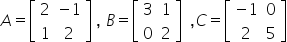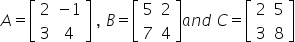Question Pool Matrices

# Matrices - SAMAGRA Question Pool & Answers | Class 12

Kerala Syllabus SAMAGRA SCERT SAMAGRA Question Pool for Class 12 Maths MatricesQn 1.

LetShow that (i) A(BC) = (AB)C

(ii) A(B+C) = AB + AC

Get Free Study Materials + 1 Week Free Trial of BrainsPrep Class 12 Tuition

Qn 2.

If(a) Prove that M(X).M(Y)=M(X+Y)

(b)Change the order as 2 x 2 by eliminating one row and one column such that M(X).M(Y)=M(X+Y)

Get Free Study Materials + 1 Week Free Trial of BrainsPrep Class 12 Tuition

Qn 3.

Letfind a matrix D such that CD = AB